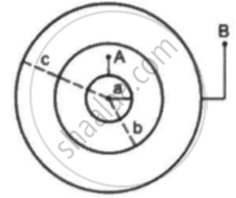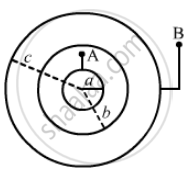Department of Pre-University Education, Karnataka course PUC Karnataka Science Class 12
Share

# Consider an Assembly of Three Conducting Concentric Spherical Shell of Radii A, B and Cas Shown in Figure Find the Capacitance of the Assembly Between the Points Aand B. - Physics

ConceptCapacitors and Capacitance

#### Question

Consider an assembly of three conducting concentric spherical shell of radii a, b and c as shown in figure Find the capacitance of the assembly between the points Aand B.#### Solution

The spherical shells form two spherical capacitors: one made by A and B and the other made by B and C.The capacitance of the spherical capacitor made by the shells of radii r1 and r2 is given by

C = (4pi∈_0)/[[1/r_1 - 1/r^2]] = (4pi∈_0r_1r_2)/(r_2 - r_1)

The capacitance of the capacitor made by A and B is given by

C_(AB) = (4pi∈_0ab)/(b-a)

The capacitance of the capacitor made by B and C is given by

C_(BC) = (4pi∈_0bc)/(c-b)

As the capacitors are in series, the net capacitance is given by

1/C = 1/C_(AB) + 1/C_(BC)

⇒ C = (C_(AB)C_(BC))/(C_(AB)+C_(BC)) = (((4pi∈_0)^2 ab^2c)/((b-a)(c-b)))/((4pi∈_0ab)/((b-a))+(4pi∈_0bc)/((c-b))

⇒ C = ((4pi∈_0ab^2c)/((b-a)(c-b)))/(((ab(c-b)+bc(b-a))/((b-a)(c-b))))

⇒ C = (4pi∈_0ab^2c)/[[ab(c-b)+bc(b-a)]]

⇒ C = (4pi∈_0ab^2c)/(b^2(c-a)) = (4pi∈_0ac)/((c-a))

Is there an error in this question or solution?

#### Video TutorialsVIEW ALL 

Solution Consider an Assembly of Three Conducting Concentric Spherical Shell of Radii A, B and Cas Shown in Figure Find the Capacitance of the Assembly Between the Points Aand B. Concept: Capacitors and Capacitance.
S